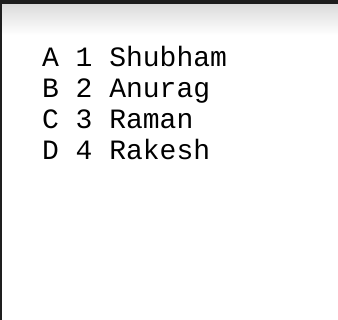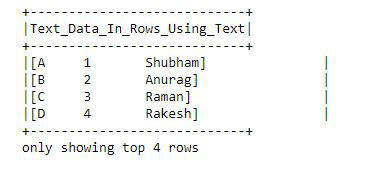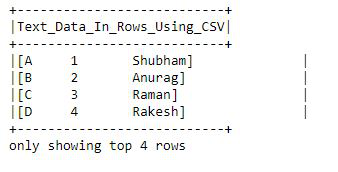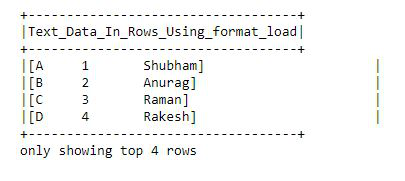Related Articles

# Read Text file into PySpark Dataframe

• Last Updated : 18 Jul, 2021

There are three ways to read text files into PySpark DataFrame.

Using these we can read a single text file, multiple files, and all files from a directory into Spark DataFrame and Dataset.

Text file Used:It is used to load text files into DataFrame whose schema starts with a string column. Each line in the text file is a new row in the resulting DataFrame. Using this method we can also read multiple files at a time.

Parameters: This method accepts the following parameter as mentioned above and described below.

• paths: It is a string, or list of strings, for input path(s).

Returns: DataFrame

Here we will import the module and create a spark session and then read the file with spark.read.text() then create columns and split the data from the txt file show into a dataframe.

## Python3

 `from` `pyspark.sql ``import` `SparkSession`` ` `spark ``=` `SparkSession.builder.appName(``"DataFrame"``).getOrCreate()`` ` `df ``=` `spark.read.text(``"output.txt"``)`` ` `df.selectExpr("split(value, ``' '``) as\``Text_Data_In_Rows_Using_Text").show(``4``,``False``)`

Output:It is used to load text files into DataFrame. Using this method we will go through the input once to determine the input schema if inferSchema is enabled. To avoid going through the entire data once, disable inferSchema option or specify the schema explicitly using the schema.

Returns: DataFrame

First, import the modules and create a spark session and then read the file with spark.read.csv(), then create columns and split the data from the txt file show into a dataframe.

## Python3

 `from` `pyspark.sql ``import` `SparkSession`` ` `spark ``=` `SparkSession.builder.getOrCreate()`` ` `df ``=` `spark.read.csv(``"output.txt"``)`` ` `df.selectExpr("split(_c0, ``' '``)\``as Text_Data_In_Rows_Using_CSV").show(``4``,``False``)`

Output:It is used to load text files into DataFrame. The .format() specifies the input data source format as “text”. The .load() loads data from a data source and returns DataFrame.

Parameters: This method accepts the following parameter as mentioned above and described below.

• paths : It is a string, or list of strings, for input path(s).
• format : It is an optional string for format of the data source. Default to ‘parquet’.
• schema : It is an optional pyspark.sql.types.StructType for the input schema.
• options : all other string options

Returns: DataFrame

First, import the modules and create a spark session and then read the file with spark.read.format(), then create columns and split the data from the txt file show into a dataframe.

## Python3

 `from` `pyspark.sql ``import` `SparkSession`` ` `spark ``=` `SparkSession.builder.getOrCreate()`` ` `df ``=` `spark.read.``format``(``"text"``).load(``"output.txt"``)`` ` `df.selectExpr("split(value, ``' '``)\``as Text_Data_In_Rows_Using_format_load").show(``4``,``False``)`

Output:Attention geek! Strengthen your foundations with the Python Programming Foundation Course and learn the basics.

To begin with, your interview preparations Enhance your Data Structures concepts with the Python DS Course. And to begin with your Machine Learning Journey, join the Machine Learning – Basic Level Course

My Personal Notes arrow_drop_up